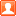# PHP单例模式应用示例【多次连接数据库只实例化一次】发表于 2019-01-05 07:57 | 只看该作者 | 倒序浏览
 本文实例讲述了PHP单例模式应用。分享给大家供大家参考，具体如下： 以前刚开始工作的时候经常连接数据库，每次用到数据库的时候就要用new进行实例并连接一次，当时因为连接数据库的次数不是很频繁，所以也没什么。后来主管对我说我现在这样每次都连接数据库的如果数据读取频繁的话对数据库和系统造成的压力会很大，让我想想办法能不能就连接一次数据库然后再次用到的时候就不用new一个新的连接了，当时怎么也没想到好的办法，知道最近学到了单例模式才恍然大悟，当时主管是引导我用单例模式的，只怪我以前对开发模式不懂。好了废话少说，下面来看单例模式： 单例模式（职责模式）： 简单的说，一个对象（在学习设计模式之前，需要比较了解面向对象思想）只负责一个特定的任务； 单例类： 1、构造函数需要标记为`private`（访问控制：防止外部代码使用new操作符创建对象），单例类不能在其他类中实例化，只能被其自身实例化； 2、拥有一个保存类的实例的静态成员变量 3、拥有一个访问这个实例的公共的静态方法（常用`getInstance()`方法进行实例化单例类，通过`instanceof`操作符可以检测到类是否已经被实例化） 另外，需要创建`__clone()`方法防止对象被复制（克隆） 为什么要使用PHP单例模式？ 1、php的应用主要在于数据库应用, 所以一个应用中会存在大量的数据库操作, 使用单例模式, 则可以避免大量的`new` 操作消耗的资源。 2、如果系统中需要有一个类来全局控制某些配置信息, 那么使用单例模式可以很方便的实现. 这个可以参看ZF的FrontController部分。 3、在一次页面请求中, 便于进行调试, 因为所有的代码(例如数据库操作类db)都集中在一个类中, 我们可以在类中设置钩子, 输出日志，从而避免到处`var_dump`, `echo`。 代码（官方文档中的）： ```bark(); // 复制对象将导致一个E_USER_ERROR. \$test_clone= clone \$test; ?> ``` 结果： I am constructed!   Woof! Fatal error: Clone is not allowed. in E:\APMServ5.2.6\www\htdocs\Lee\myprogram\other\class\singletons.phpon line 31 以下内容源自网络（可以参考学习一下）： 单例模式的三个要点： （1）. 需要一个保存类的唯一实例的静态成员变量:   ```private static \$_instance; ``` （2）. 构造函数和克隆函数必须声明为私有的，防止外部程序new类从而失去单例模式的意义: ```private function __construct() { \$this->_db = pg_connect('xxxx'); } private function __clone() { }//覆盖__clone()方法，禁止克隆 ``` （3）. 必须提供一个访问这个实例的公共的静态方法（通常为`getInstance`方法），从而返回唯一实例的一个引用 ```public static function getInstance() { if(! (self::\$_instance instanceof self) ) { self::\$_instance = new self(); } return self::\$_instance; } ``` 二、为什么要使用单例模式？ 1、PHP缺点： PHP语言是一种解释型的脚本语言，这种运行机制使得每个PHP页面被解释执行后，所有的相关资源都会被回收。也就是说，PHP在语言级别上没有办法让某个对象常驻内存，这和asp.NET、Java等编译型是不同的，比如在Java中单例会一直存在于整个应用程序的生命周期里，变量是跨页面级的，真正可以做到这个实例在应用程序生命周期中的唯一性。然而在PHP中，所有的变量无论是全局变量还是类的静态成员，都是页面级的，每次页面被执行时，都会重新建立新的对象，都会在页面执行完毕后被清空，这样似乎PHP单例模式就没有什么意义了，所以PHP单例模式我觉得只是针对单次页面级请求时出现多个应用场景并需要共享同一对象资源时是非常有意义的。 2、单例模式在PHP中的应用场合： （1）、应用程序与数据库交互 一个应用中会存在大量的数据库操作，比如过数据库句柄来连接数据库这一行为，使用单例模式可以避免大量的new操作，因为每一次`new`操作都会消耗内存资源和系统资源。 （2）、控制配置信息 如果系统中需要有一个类来全局控制某些配置信息,那么使用单例模式可以很方便的实现. 三、如何实现单例模式？ 1、普通的数据库访问例子： ```addUserInfo(...); ...... //在函数中访问数据库，查找用户信息 function getUserInfo() { \$db = new DB(...);//再次new 数据库类，和数据库建立连接 \$db = query(....);//根据查询语句访问数据库 } ?> ``` 2、应用单例模式对数据库进行操作： ```_db = pg_connect(...);//postgrsql } private function __clone() {}; //覆盖__clone()方法，禁止克隆 public static function getInstance() { if(! (self::\$_instance instanceof self) ) { self::\$_instance = new self(); } return self::\$_instance; } public function addUserInfo(...) { } public function getUserInfo(...) { } } //test \$db = DB::getInstance(); \$db->addUserInfo(...); \$db->getUserInfo(...); ?> ``` 3、深入理解 ```conn = mysql_connect(\$db['host'],\$db['user'],\$db['password']); if(!mysql_select_db(\$db['database'],\$this->conn)){ echo "失败"; }; mysql_query('set names utf8',\$this->conn); } public static function getInstance(){ if(is_null(self::\$instance)){ self::\$instance = new db; } return self::\$instance; } /** * 查询数据库 */ public function select(\$table,\$condition=array(),\$field = array()){ \$where=''; if(!empty(\$condition)){ foreach(\$condition as \$k=>\$v){ \$where.=\$k."='".\$v."' and "; } \$where='where '.\$where .'1=1'; } \$fieldstr = ''; if(!empty(\$field)){ foreach(\$field as \$k=>\$v){ \$fieldstr.= \$v.','; } \$fieldstr = rtrim(\$fieldstr,','); }else{ \$fieldstr = '*'; } self::\$sql = "select {\$fieldstr} from {\$table} {\$where}"; \$result=mysql_query(self::\$sql,\$this->conn); \$resuleRow = array(); \$i = 0; while(\$row=mysql_fetch_assoc(\$result)){ foreach(\$row as \$k=>\$v){ \$resuleRow[\$i][\$k] = \$v; } \$i++; } return \$resuleRow; } /** * 添加一条记录 */ public function insert(\$table,\$data){ \$values = ''; \$datas = ''; foreach(\$data as \$k=>\$v){ \$values.=\$k.','; \$datas.="'\$v'".','; } \$values = rtrim(\$values,','); \$datas = rtrim(\$datas,','); self::\$sql = "INSERT INTO {\$table} ({\$values}) VALUES ({\$datas})"; if(mysql_query(self::\$sql)){ return mysql_insert_id(); }else{ return false; }; } /** * 修改一条记录 */ public function update(\$table,\$data,\$condition=array()){ \$where=''; if(!empty(\$condition)){ foreach(\$condition as \$k=>\$v){ \$where.=\$k."='".\$v."' and "; } \$where='where '.\$where .'1=1'; } \$updatastr = ''; if(!empty(\$data)){ foreach(\$data as \$k=>\$v){ \$updatastr.= \$k."='".\$v."',"; } \$updatastr = 'set '.rtrim(\$updatastr,','); } self::\$sql = "update {\$table} {\$updatastr} {\$where}"; return mysql_query(self::\$sql); } /** * 删除记录 */ public function delete(\$table,\$condition){ \$where=''; if(!empty(\$condition)){ foreach(\$condition as \$k=>\$v){ \$where.=\$k."='".\$v."' and "; } \$where='where '.\$where .'1=1'; } self::\$sql = "delete from {\$table} {\$where}"; return mysql_query(self::\$sql); } public static function getLastSql(){ echo self::\$sql; } } \$db = db::getInstance(); //\$list = \$db->select('demo',array('name'=>'tom','password'=>'ds'),array('name','password')); //echo \$db->insert('demo',array('name'=>'最近你啦','password'=>'123')); //echo \$db->update('demo',array("name"=>'xxx',"password"=>'123'),array('id'=>1)); echo \$db->delete('demo',array('id'=>'2')); db::getLastSql(); echo "
"; ?>  ```  更多关于PHP相关内容感兴趣的读者可查看本站专题：《php面向对象程序设计入门教程》、《PHP数组(Array)操作技巧大全》、《PHP基本语法入门教程》、《PHP运算与运算符用法总结》、《php字符串(string)用法总结》、《php+mysql数据库操作入门教程》及《php常见数据库操作技巧汇总》 希望本文所述对大家PHP程序设计有所帮助。